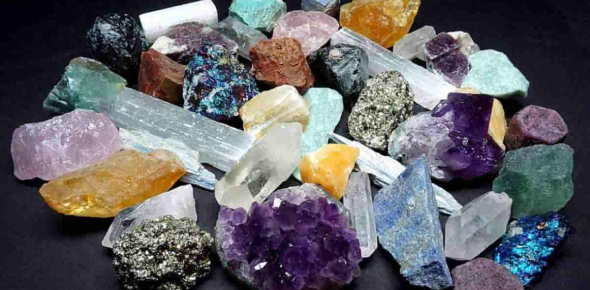# Rocks And Minerals Quiz: MCQ! Trivia

21 Questions | Attempts: 191
ShareSettings.

• 1.
The words waxy, pearly, and dull describe a mineral's...
• A.

Luster

• B.

Hardness

• C.

Fracture

• D.

Cleavage

• 2.
The words uneven and splintery describe a mineral's:
• A.

Luster

• B.

Hardness

• C.

Fracture

• D.

Cleavage

• 3.
The ratio of a mineral's mass to its volume is the mineral's:
• A.

Atomic weight

• B.

Density

• C.

Mass

• D.

Weight

• 4.
Non-Silicates are the most abundant group of minerals in the Earth's crust.
• A.

True

• B.

False

• 5.
Minerals that have the "closest packing" structure have high densities.
• A.

True

• B.

False

• 6.
The tetrahedral arrangement of atoms is found only in silicate minerals.
• A.

True

• B.

False

• 7.
The splitting of slate into flat layers illustrates its:
• A.

Foliation

• B.

Sediment

• C.

Formation

• D.

Contact metamorphism

• 8.
Partial melting is when the different minerals in rock have different melting points.
• A.

True

• B.

False

• 9.
Fractional crystallization is when the minerals in a rock crystallize at different temperatures.
• A.

True

• B.

False

• 10.
What belongs in space A?
• A.

Metamorphic Rock

• B.

Sedimentary Rock

• C.

Igneous Rock

• 11.
What belongs in space B?
• A.

Sedimentary Rock

• B.

Metamorphic Rock

• C.

Igneous Rock

• 12.
What belongs in space C?
• A.

Sedimentary Rock

• B.

Metamorphic Rock

• C.

Igneous Rock

• 13.
The gram formula weight (weight of one mole) of the mineral quartz is 44 g, and the gram formula weight of magnetite is 136 g.  If you had 4 moles of magnetite, how many moles of quartz would be equal to the weight of the magnetite?  (Round to the nearest whole number)
• A.

10 moles

• B.

15 moles

• C.

12 moles

• D.

3 moles

• 14.
Rocks are classified into three categories: sedimentary, metamorphic, and igneous.
• A.

True

• B.

False

• 15.
The gram formula weight (weight of one mole) of the mineral hematite, is 285 g, and the gram formula weight of magnetite is 345 g.  Which of the following would weigh more: half a mole of hematite or one-third of a mole of magnetite?
• A.

Hematite

• B.

Magnetite

• 16.
Which of the following measurements represent mass?
• A.

Grams

• B.

Centimeters cubed

• C.

Density

• D.

None of the above

• 17.
Which of the following is NOT a characteristic a substance must have to be classified as a mineral?
• A.

Inorganic

• B.

Crystalline solid

• C.

Organic

• D.

Occurs naturally

• E.

Consistent chemical composition

• 18.
Magma that solidifies underground forms rock masses that are known as...
• A.

Lava plateaus

• B.

Intrusions

• C.

Extrusions

• D.

Volcanic cones

• 19.
Light-colored igneous rocks are generally part of the:
• A.

Intermediate family

• B.

Mafic family

• C.

Felsic family

• D.

Basalt family

• 20.
When two single chains of tetrahedra bond to each other, the result is called a?
• A.

Double-chain silicate

• B.

Sheet silicate

• C.

Single-chain silicate

• 21.
How many oxygen ions and silicon ions are in a silicon-oxygen tetrahedron?
• A.

4 oxygen, 1 silicon

• B.

1 silicon, 4 oxygen

• C.

3 oxygen, 1 silicon

• D.

1 oxygen, 3 silicon

## Related TopicsBack to top
×

Wait!
Here's an interesting quiz for you.